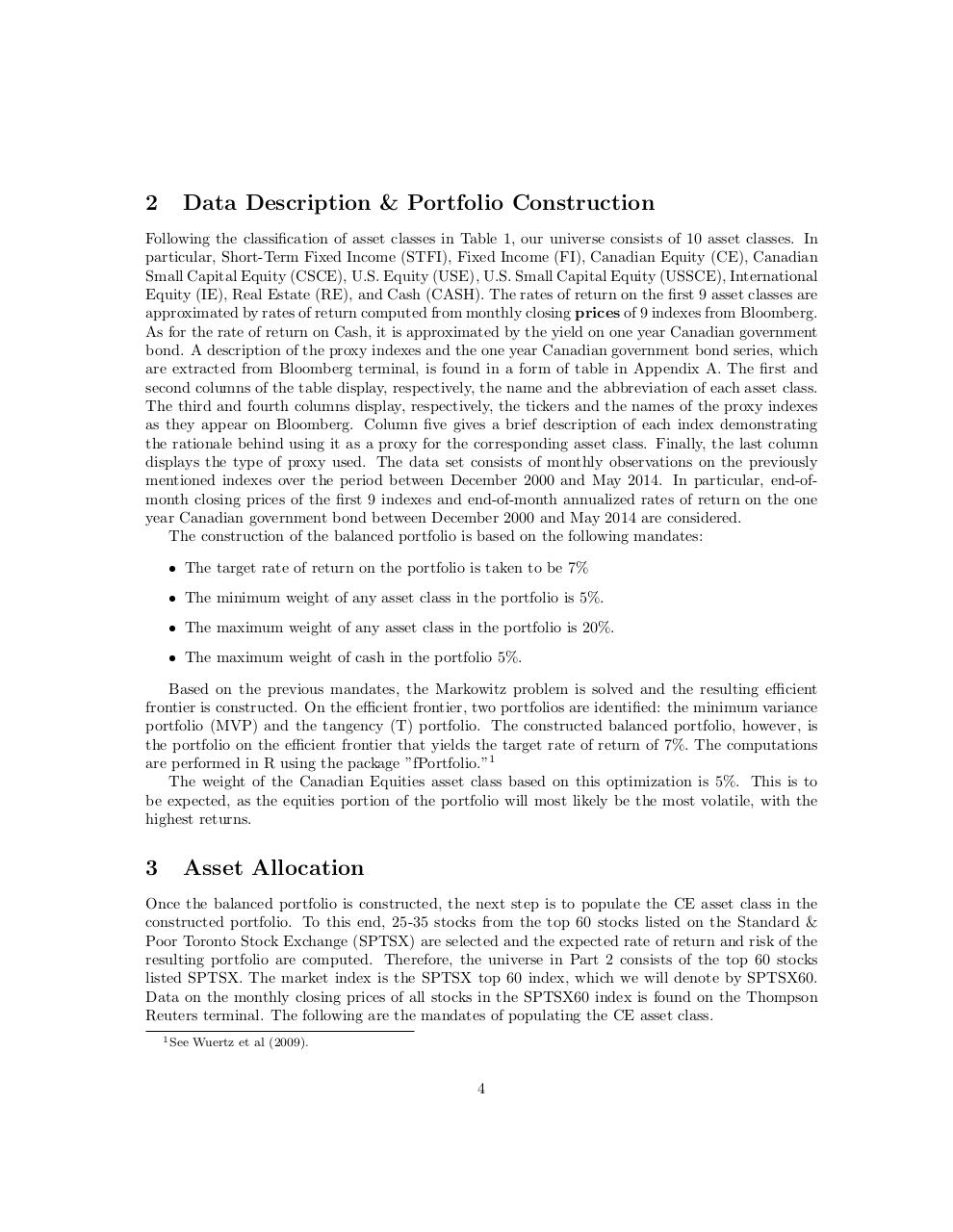# Workshop Draft.pdfPage 1 2 3 45622

#### Text preview

2

Data Description &amp; Portfolio Construction

Following the classification of asset classes in Table 1, our universe consists of 10 asset classes. In
particular, Short-Term Fixed Income (STFI), Fixed Income (FI), Canadian Equity (CE), Canadian
Small Capital Equity (CSCE), U.S. Equity (USE), U.S. Small Capital Equity (USSCE), International
Equity (IE), Real Estate (RE), and Cash (CASH). The rates of return on the first 9 asset classes are
approximated by rates of return computed from monthly closing prices of 9 indexes from Bloomberg.
As for the rate of return on Cash, it is approximated by the yield on one year Canadian government
bond. A description of the proxy indexes and the one year Canadian government bond series, which
are extracted from Bloomberg terminal, is found in a form of table in Appendix A. The first and
second columns of the table display, respectively, the name and the abbreviation of each asset class.
The third and fourth columns display, respectively, the tickers and the names of the proxy indexes
as they appear on Bloomberg. Column five gives a brief description of each index demonstrating
the rationale behind using it as a proxy for the corresponding asset class. Finally, the last column
displays the type of proxy used. The data set consists of monthly observations on the previously
mentioned indexes over the period between December 2000 and May 2014. In particular, end-ofmonth closing prices of the first 9 indexes and end-of-month annualized rates of return on the one
year Canadian government bond between December 2000 and May 2014 are considered.
The construction of the balanced portfolio is based on the following mandates:
• The target rate of return on the portfolio is taken to be 7%
• The minimum weight of any asset class in the portfolio is 5%.
• The maximum weight of any asset class in the portfolio is 20%.
• The maximum weight of cash in the portfolio 5%.
Based on the previous mandates, the Markowitz problem is solved and the resulting efficient
frontier is constructed. On the efficient frontier, two portfolios are identified: the minimum variance
portfolio (MVP) and the tangency (T) portfolio. The constructed balanced portfolio, however, is
the portfolio on the efficient frontier that yields the target rate of return of 7%. The computations
are performed in R using the package ”fPortfolio.”1
The weight of the Canadian Equities asset class based on this optimization is 5%. This is to
be expected, as the equities portion of the portfolio will most likely be the most volatile, with the
highest returns.

3

Asset Allocation

Once the balanced portfolio is constructed, the next step is to populate the CE asset class in the
constructed portfolio. To this end, 25-35 stocks from the top 60 stocks listed on the Standard &amp;
Poor Toronto Stock Exchange (SPTSX) are selected and the expected rate of return and risk of the
resulting portfolio are computed. Therefore, the universe in Part 2 consists of the top 60 stocks
listed SPTSX. The market index is the SPTSX top 60 index, which we will denote by SPTSX60.
Data on the monthly closing prices of all stocks in the SPTSX60 index is found on the Thompson
Reuters terminal. The following are the mandates of populating the CE asset class.
1 See

Wuertz et al (2009).

4